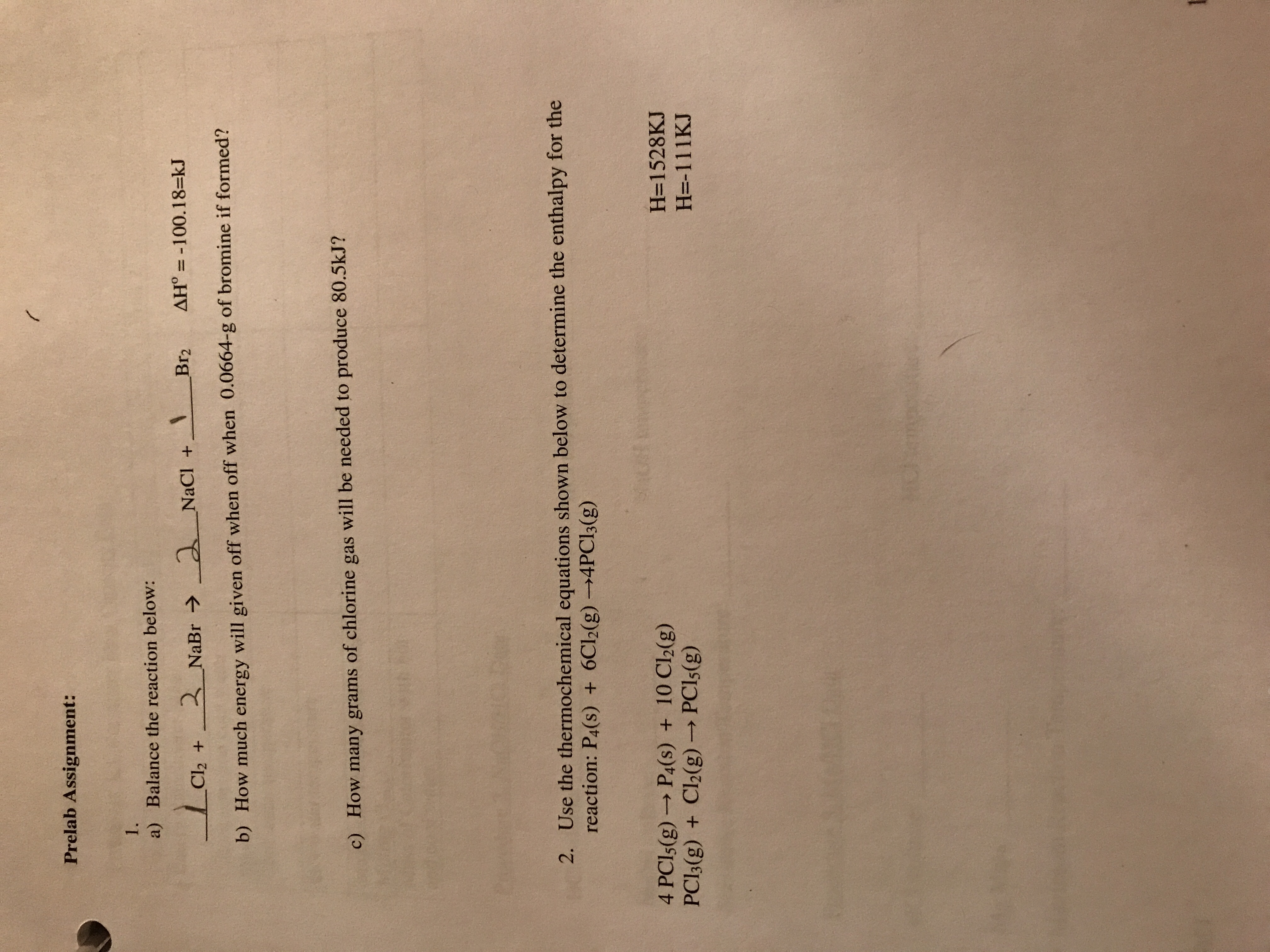# Prelab Assignment: a) Balance the reaction below: BT2 ΔΗ'--100.18 kJ + b) How much energy will given off when off when 0.0664-g of bromine if formed? c) How many grams of chlorine gas will be needed to produce 80.5kJ? Use the thermochemical equations shown below to determine the enthalpy for the reaction: P4(s) + 6C12(g)--4PC13(g) 2. 4 PCIs(g)- P4(s) + 10 Cl2(g) PCI3(g) + Cl2(g) → PC15(g) H-1528KJ H=-111KJ

Question

Questions in photo.help_outlineImage TranscriptionclosePrelab Assignment: a) Balance the reaction below: BT2 ΔΗ'--100.18 kJ + b) How much energy will given off when off when 0.0664-g of bromine if formed? c) How many grams of chlorine gas will be needed to produce 80.5kJ? Use the thermochemical equations shown below to determine the enthalpy for the reaction: P4(s) + 6C12(g)--4PC13(g) 2. 4 PCIs(g)- P4(s) + 10 Cl2(g) PCI3(g) + Cl2(g) → PC15(g) H-1528KJ H=-111KJ fullscreen